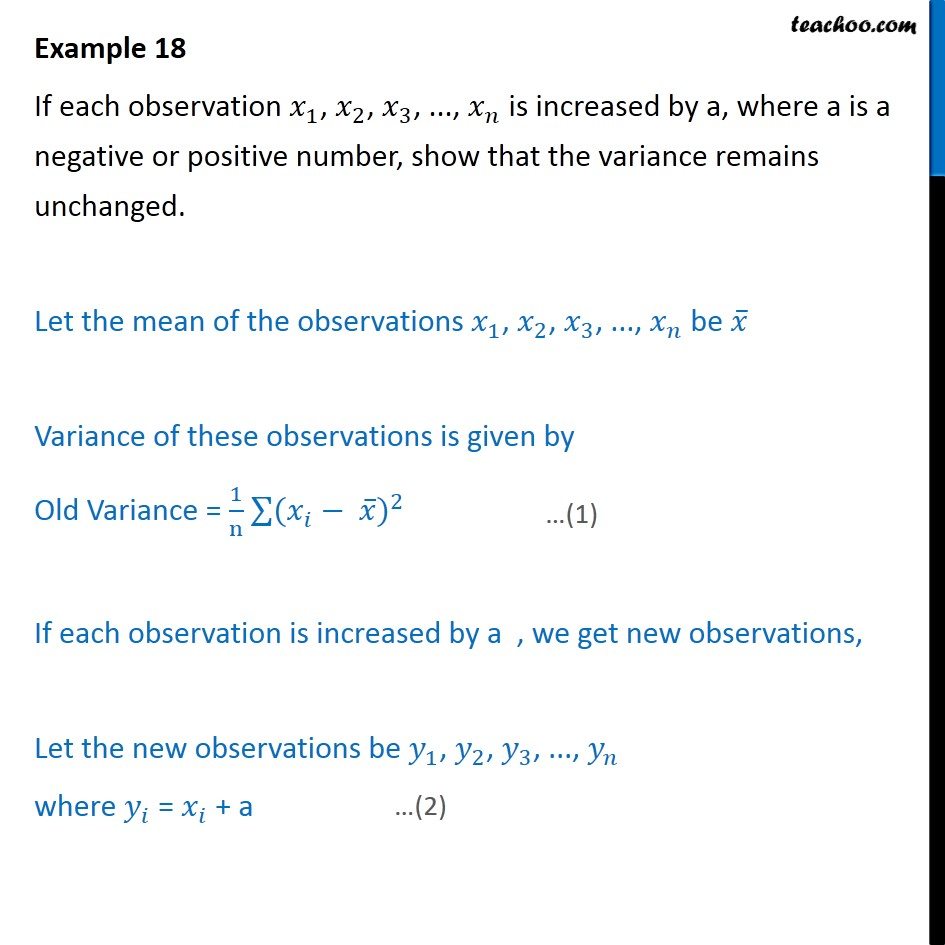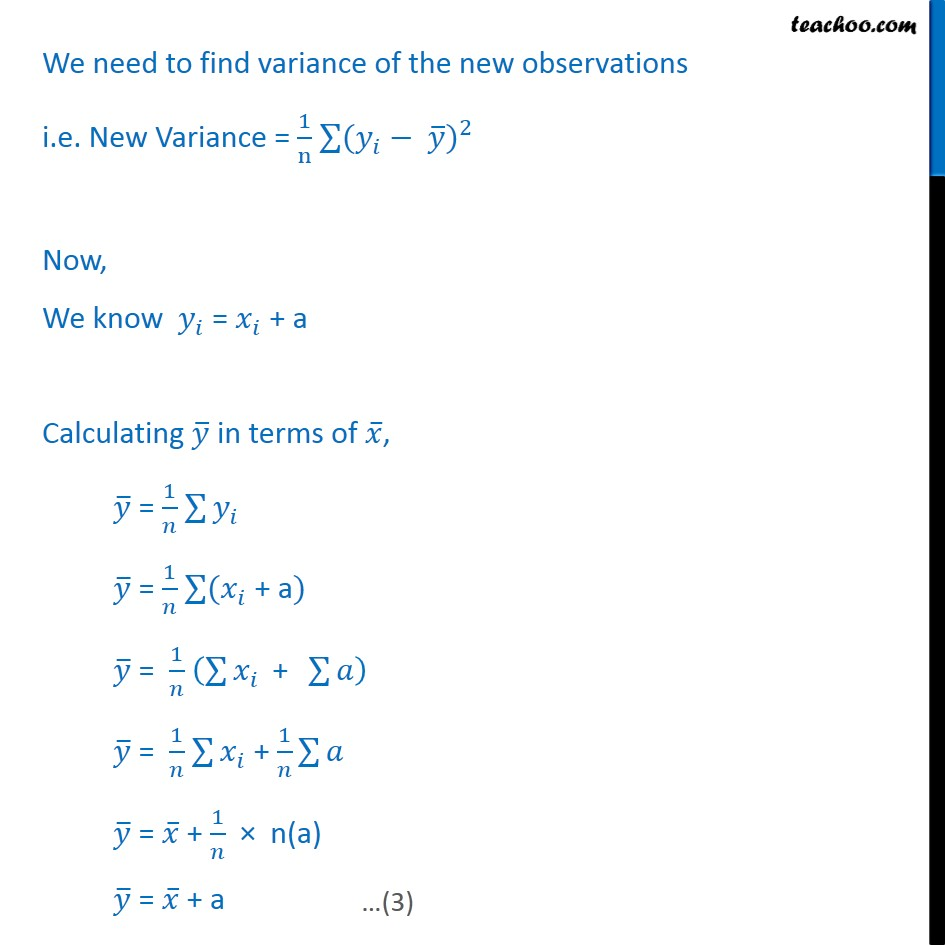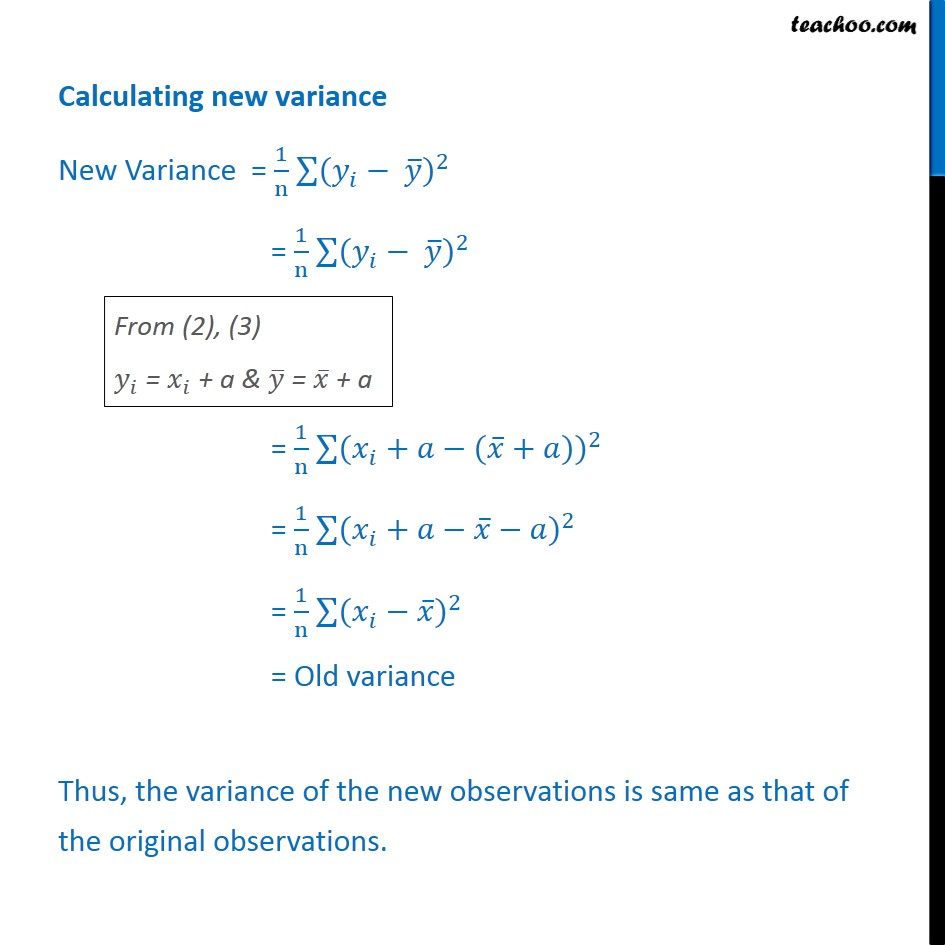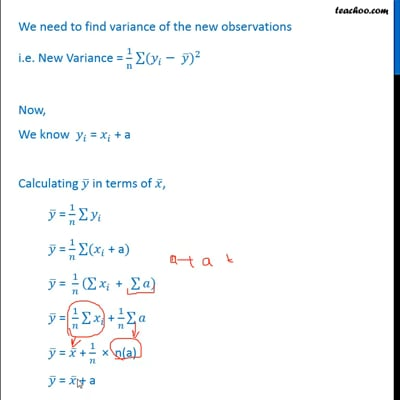Examples

Chapter 15 Class 11 Statistics (Term 1)
Serial order wiseThis video is only available for Teachoo black users

### Transcript

Example 18 If each observation 𝑥﷮1﷯, 𝑥﷮2﷯, 𝑥﷮3﷯, ..., 𝑥﷮𝑛﷯ is increased by a, where a is a negative or positive number, show that the variance remains unchanged. Let the mean of the observations 𝑥﷮1﷯, 𝑥﷮2﷯, 𝑥﷮3﷯, ..., 𝑥﷮𝑛﷯ be 𝑥﷯ Variance of these observations is given by Old Variance = 1﷮n﷯ ﷮﷮( 𝑥﷮𝑖﷯﷯− 𝑥﷯)﷮2﷯ If each observation is increased by a , we get new observations, Let the new observations be 𝑦﷮1﷯, 𝑦﷮2﷯, 𝑦﷮3﷯, ..., 𝑦﷮𝑛﷯ where 𝑦﷮𝑖﷯ = 𝑥﷮𝑖﷯ + a We need to find variance of the new observations i.e. New Variance = 1﷮n﷯ ﷮﷮( 𝑦﷮𝑖﷯﷯− 𝑦﷯)﷮2﷯ Now, We know 𝑦﷮𝑖﷯ = 𝑥﷮𝑖﷯ + a Calculating 𝑦﷯ in terms of 𝑥﷯, 𝑦﷯ = 1﷮𝑛﷯ ﷮﷮ 𝑦﷮𝑖﷯﷯ 𝑦﷯ = 1﷮𝑛﷯ ﷮﷮( 𝑥﷮𝑖﷯﷯ + a) 𝑦﷯ = 1﷮𝑛﷯ ﷮﷮ 𝑥﷮𝑖﷯﷯ + ﷮﷮𝑎﷯﷯ 𝑦﷯ = 1﷮𝑛﷯ ﷮﷮ 𝑥﷮𝑖﷯﷯ + 1﷮𝑛﷯ ﷮﷮𝑎﷯ 𝑦﷯ = 𝑥﷯ + 1﷮𝑛﷯ × n(a) 𝑦﷯ = 𝑥﷯ + a Calculating new variance New Variance = 1﷮n﷯ ﷮﷮( 𝑦﷮𝑖﷯﷯− 𝑦﷯)﷮2﷯ = 1﷮n﷯ ﷮﷮( 𝑦﷮𝑖﷯﷯− 𝑦﷯)﷮2﷯ = 1﷮n﷯ ﷮﷮( 𝑥﷮𝑖﷯+𝑎﷯−( 𝑥﷯+𝑎))﷮2﷯ = 1﷮n﷯ ﷮﷮( 𝑥﷮𝑖﷯+𝑎﷯− 𝑥﷯−𝑎)﷮2﷯ = 1﷮n﷯ ﷮﷮( 𝑥﷮𝑖﷯﷯− 𝑥﷯)﷮2﷯ = Old variance Thus, the variance of the new observations is same as that of the original observations.